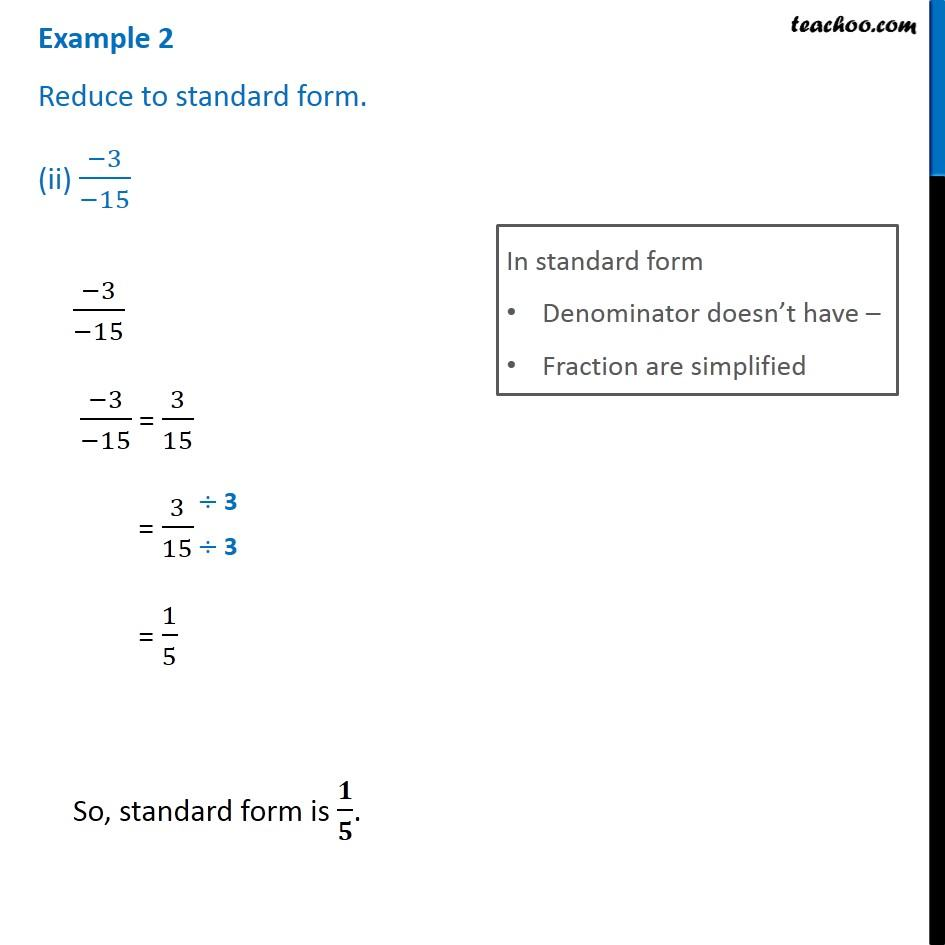Examples

Chapter 8 Class 7 Rational Numbers
Serial order wiseLearn in your speed, with individual attention - Teachoo Maths 1-on-1 Class

### Transcript

Example 2 Reduce to standard form. (ii) (−3)/(−15) In standard form Denominator doesn’t have – Fraction are simplified (−3)/(−15) (−3)/(−15) = 3/15 = 3/15 = 1/5 So, standard form is 𝟏/𝟓.